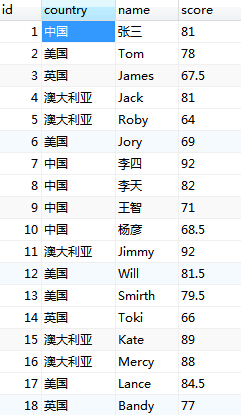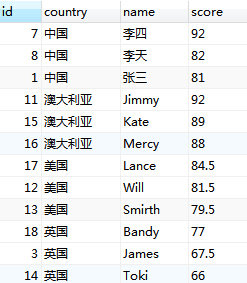# 分组查询取每组前n条记录实例1、

select t3.id,t3.country,t3.score
from (select t1.*, (select count(*) from tb_score t2 where t1.score<=t2.score and t1.country=t2.country) as rownum
from tb_score t1) t3
where rownum <=3 order by country,score DESC;

2、

select a.id,a.country,a.name,a.score from tb_score a
where exists
(select count(*) from tb_score where country = a.country and score > a.score having Count(*) < 3)
order by a.country,score DESC;

3、

select a.id,a.country,a.name,a.score from tb_score a
where
(select count(*) from tb_score where country = a.country and score > a.score )<3
order by a.country,score DESC;